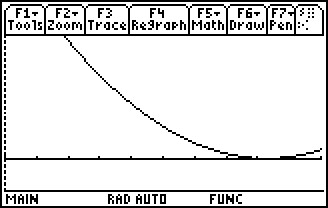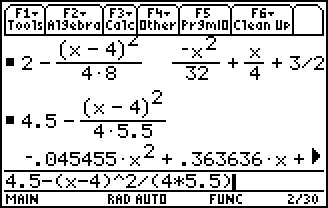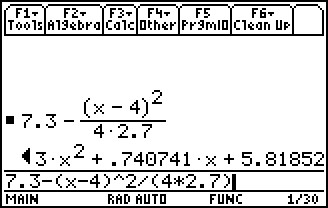# Activities

••• ##### Subject Area

• Math: Calculus: Applications of Integral, Antiderivatives and Definite Integrals

• ##### Author9-12

45 Minutes

• ##### Device
• TI-89 / TI-89 Titanium

## Torricelli's Law#### Activity Overview

Students will examine the velocity of water flowing through the tap of a tank, finding an equation to model the height of the water in the tank as the tank is drained.

#### Key Steps

•Students use the principle of conservation of energy, to discuss that potential energy possessed by water is to the top of the tank, the kinetic energy as it leaves through the tap, and then equate these to derive the common form for Torricelli’s Law.

•Students then make a connection between the equation given by Torricelli’s law and a differential equation for the change in volume with respect to time. They identify all the relevant parameters and functions, which apply to such a system, and how these relate to each other. Students build a relationship between time and height, which leads to a formula for height with respect to time.

•Students will describe this situation as a graphical representation of height vs. time. At the end of this activity, given a function that expresses the velocity or acceleration of a moving object as a function of time, students integrate to find a function that describes the displacement as a function of time.